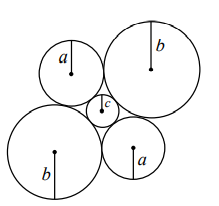Close

# 「數學」好好做着幾何題，怎麼就變數論了呢？

Everything starts with an innocent-looking question from the last question of 2021 Euclid contest:## Question

Suppose that c is a positive integer. Define f(c) to be the number of pairs (a,b) with c < a < b for wich two circles of radius a, two circles of radius b, and one circle of radius c can be drawn so that

• each circle of radius a is tangent to both circles of radius b and to the circle of radius c, and
• each circle of radius b is tangent to both circles of radius a and to the circle of radius c,

as shown, Determine all positive interger c for which f(c) is even.

## Solution (Part 1)

First it is easy to infer that the centres of the outside circles form the vertices of a rhombus. Hence, the centres of any three adjacent circles form a right-angled triangle, with three sides of a+c, b+c, and a+b. Pythagoras Theorem automatically results:

(a+c)^2+(b+c)^2=(a+b)^2\\(a-c)(b-c)=2c^2

The inequality between a, b, and c ensures that a-c\ne b-c. The problem now becomes:

Find such c that the number 2c^2 can be expressed as the product of two integers in even number of ways.

### [Detour] Ways of decompose an integer

For any natural number that is not a perfect square, its factors always come in pairs, since if p|c then we must have \frac cp|c. As a result of this, for a non-squared number c, there are always \frac n2 ways to decompose it, where n is the number of factors of c.

### [Detour] Number of factors of a natural number

Fundamental Theory of Arithmetic states that the prime factor decomposition is unique for every natural number. Hence, assume that:

c=\prod\limits_ip_i^{e_i}\in\mathbb{N}.

Consider the ith prime factor, it can have e_i+1 states, namely: \{0, 1, \ldots, e_i\}. Therefore, there will be K factors of c where

K=\prod_i(e_i+1).

## Solution (Part 2)

Now that we know there should be even ways to decompose 2c^2, assume that c=2^k\prod\limits_ip_i^{e_i}, then:

2c^2=2^{2k+1}\prod_ip_i^{2e_i},\\K_{2c^2}=(2k+2)\prod_i(2e_i+1)

That is, for any c=2^k\prod\limits_ip_i^{e_i}, there are f(c)=\frac{K_{2c^2}}2=(k+1)\prod\limits_i(2e_i+1) ways of decomposing 2c^2. Since 2e_i+1\equiv1\pmod2, f(c)\equiv0\pmod2\iff k\equiv1\pmod2

## Conclusion

To make f(c) even, c has to have the following prime factor decomposition:

## 心路歷程

### Pythagorean Tuple

Given that the three sides of a right-angled triangle are all integers: (a, b, c)\in\mathbb{Z}^3, then the tuple is referred to as Pythagorean tuple. Pythagorean tuples can be generated by two integers u, v:

a=u^2-v^2,\\b=2uv,\\c=u^2+v^2.

This site uses Akismet to reduce spam. Learn how your comment data is processed.